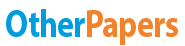# Case 19 Stanley Products

Essay by   •  July 12, 2019  •  Case Study  •  909 Words (4 Pages)  •  597 Views

## Essay Preview: Case 19 Stanley Products

Report this essay
Page 1 of 4

Given Terms:

2/10, net 30

70% will pay using discount--10 day

25% will pay without discount, but on time--30 days

5% will pay late--50 days

Increase in sales is 10%

Variable Cost is 70%

Cost of Capital is 5%

R is ???

Q1. SP’s bad debt is pretty high compared to average bad debt expense of other companies. Stanley Products need to tighten their credit terms, which will get rid of customers who are unable to pay without a discount and/or on time, and keep existing clients who can pay for purchases almost right away. This will reduce bad debt expenses.

Q2.

A)T+1Sales: \$987 x 1.10 = \$1,085.7

A/S: \$361.4/\$987 = \$.366

L/S: (\$45.6 + \$39.7)/\$987 = \$.086

N= A/S - L/S⇒ \$.366 - \$.086 = \$.28

Change in Sales: \$1,400.2 - \$987 = \$413.2

NI/Sales: 34.3/987 = .035 = 3.5% Net Profit Margin

EFR = .28(413.2) - .035(1,400.2)

EFR = 115.70 - 49.00

EFR = \$66.7 need to finance

T+2 Sales: \$1,400.2 x 1.10 = \$1,540.22

Inventory and Receivables increased by 12%:

(123.4 x 12%) + (109.7 x 12%) + 361.4 = 389.37 new total assets

A/S: \$389.37/\$1,400.2 = \$.278

L/S: (\$45.6 + \$39.7)/\$1,400.2 = \$.061

N= A/S - L/S⇒ \$.278 - \$.061 = \$.217

Change in Sales: \$1,778.3 - \$1,400.2 = \$378.1

 Sales \$1,400.20 Bad Debt Expense \$7.00 (\$1,400.2 x .005) Net Sales \$1,393.20 Variable Cost \$980.14 (\$1,400.2 x .70) Fixed Cost \$176.70 Earnings before taxes \$236.36 Taxes (40%) \$95.54 Net Income \$141.82

NI/Sales: 141.82/1,400.2 = .1013 = 10.13% Net Profit Margin

EFR = .217(378.1) - .1013(1,778.3)

EFR = 82.05- 180.14

EFR = 98.09

T+3 Sales: \$1,778.3 x 1.10 = \$1,956.13

Inventory and Receivables increased by 12%:

(138.21 x 12%) +( 122.86 x 12% ) + 389.37 = 420.70

A/S: \$420.70/\$1,956.13 = \$.2151

L/S: (\$45.6 + \$39.7)/\$1,956.13 = \$.044

N= A/S - L/S⇒ \$.2151 - \$.044 = \$.1711

Change in Sales: \$2,294.1 - \$1,778.3 = \$515.8

 Sales \$1,778.3 Bad Debt Expense \$8.89 (\$1,778.3 x .005) Net Sales \$1,769.41 Variable Cost \$1,244.81(\$1,778.3 x .70) Fixed Cost \$176.70 Earnings before taxes \$347.9 Taxes (40%) \$139.16 Net Income \$208.74

NI/Sales: 208.74/1,778.3 = .1174 = 11.74% Net Profit Margin

EFR = .1711(515.8)- .1174(2,294.1)

EFR = 88.25 - 269.33

EFR = 181.08

B) g = (.035)(1.00)/(.28-.035)

g= .035/.245

g= 14.30%

C)

No Growth

 T=0 T=1 T=2 T=3 \$987.0 \$1,400.2 \$1,778.3 \$2,294.1

Internal financing

 T=0 T=1 T=2 T=3 \$987.0 \$1,128.1 \$1,289.4 \$1,473.8 (987 x 1.143) (1,128.14 x 1.143) (1,289.4 x 1.143)

Q3.

Part A

1/10 net 20

Cost of Credit = Discount %/ (100-Discount %) x (360/Allowed payment days – Discount days)

Cost of Credit = 1%/ (100-1%)*(360/20-10) = 36.36%

Part B

2/10 net 30

Cost of Credit = 2%/ (100-2%)*(360/30-10) = 36.73%

Part C

3/10 net 45

Cost of Credit = 3%/ (100-3%)*(360/45-10) = 31.81%

Q4.

Part A

The average collection period = 70%*10+25%*30+5%*50 = 17 Days

Part B

EXHIBIT 3

Worksheet to Calculate Incremental Asset Requirements and Capital Cost of Credit Changes for 1997 (Year t + 1) (\$000s)

 No Credit Changes With changes Differences Investment in receivables 116.7 17 x (1540.22/360)= 72.73 x .70= 50.91 -65.79 Inventory needed 175.1 192.56 17.46 Total 291.8 243.47 -48.33 Capital Cost 35.0 12.17 -22.83

Assuming the proposed credit changes are made. Sp’s has outstanding day sales and an average collection period.

Q5.

 EXHIBIT 4 No Changes With Changes Difference Sales 1400.2 1540.22 +140.02 Bad Debt Expense 18.2 7.70 -10.5 Discounts taken 0 32.34 +32.34 Net Sales 1382.0 1500.18 +118.18 Variable Cost 1050.2 1078.15 +27.95 Fixed Cost 252.0 252.0 0 EBT 79.8 170.03 +90.23 Taxes (40%) 31.9 68.01 +36.11 Net Income 47.9 102.02 +54.12 Capital Cost 35.0 12.17 -22.83 Gain (loss) 12.9 89.85 +76.95

...

...